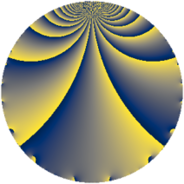# Properties

 Label 1444.1.cLevel $1444$ Weight $1$ Character orbit 1444.c Rep. character $\chi_{1444}(721,\cdot)$ Character field $\Q$ Dimension $2$ Newform subspaces $1$ Sturm bound $190$ Trace bound $0$

# Related objects

## Defining parameters

 Level: $$N$$ $$=$$ $$1444 = 2^{2} \cdot 19^{2}$$ Weight: $$k$$ $$=$$ $$1$$ Character orbit: $$[\chi]$$ $$=$$ 1444.c (of order $$2$$ and degree $$1$$) Character conductor: $$\operatorname{cond}(\chi)$$ $$=$$ $$19$$ Character field: $$\Q$$ Newform subspaces: $$1$$ Sturm bound: $$190$$ Trace bound: $$0$$

## Dimensions

The following table gives the dimensions of various subspaces of $$M_{1}(1444, [\chi])$$.

Total New Old
Modular forms 34 2 32
Cusp forms 4 2 2
Eisenstein series 30 0 30

The following table gives the dimensions of subspaces with specified projective image type.

$$D_n$$ $$A_4$$ $$S_4$$ $$A_5$$
Dimension 0 0 2 0

## Trace form

 $$2 q + 2 q^{5} + 2 q^{7} - 2 q^{9} + O(q^{10})$$ $$2 q + 2 q^{5} + 2 q^{7} - 2 q^{9} - 2 q^{11} + 2 q^{17} + 2 q^{35} - 2 q^{43} - 2 q^{45} - 2 q^{47} - 2 q^{55} - 2 q^{61} - 2 q^{63} + 2 q^{73} - 2 q^{77} - 2 q^{81} + 2 q^{85} + 4 q^{87} + 2 q^{99} + O(q^{100})$$

## Decomposition of $$S_{1}^{\mathrm{new}}(1444, [\chi])$$ into newform subspaces

Label Dim $A$ Field Image CM RM Traces $q$-expansion
$a_{2}$ $a_{3}$ $a_{5}$ $a_{7}$
1444.1.c.a $2$ $0.721$ $$\Q(\sqrt{-2})$$ $S_{4}$ None None $$0$$ $$0$$ $$2$$ $$2$$ $$q-\beta q^{3}+q^{5}+q^{7}-q^{9}-q^{11}-\beta q^{15}+\cdots$$

## Decomposition of $$S_{1}^{\mathrm{old}}(1444, [\chi])$$ into lower level spaces

$$S_{1}^{\mathrm{old}}(1444, [\chi]) \cong$$ $$S_{1}^{\mathrm{new}}(76, [\chi])$$$$^{\oplus 2}$$